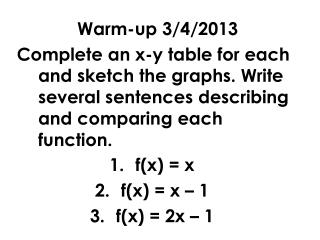DownloadDownload Presentationf(x) = x f(x) = x – 1 f(x) = 2x – 1

# f(x) = x f(x) = x – 1 f(x) = 2x – 1

Télécharger la présentation## f(x) = x f(x) = x – 1 f(x) = 2x – 1

- - - - - - - - - - - - - - - - - - - - - - - - - - - E N D - - - - - - - - - - - - - - - - - - - - - - - - - - -
##### Presentation Transcript

1. Warm-up 3/4/2013 Complete an x-y table for each and sketch the graphs. Write several sentences describing and comparing each function. • f(x) = x • f(x) = x – 1 • f(x) = 2x – 1

2. p. 73 MCC9-12.F.BF.3 Identify the effect on the graph of replacing f(x) by f(x) + k, k f(x), f(kx), and f(x + k) for specific values of k (both positive and negative); find the value of k given the graphs. Experiment with cases and illustrate an explanation of the effects on the graphusing technology. Include recognizing even and odd functionsfrom their graphs and algebraic expressions for them.

3. Transformations of linear graphs Topic: What is it? Shifting, stretching, shrinking, and reflecting of graphs Vertical or Horizontal shift Vertical Stretch or Shrink Types: Reflection Add outside Multiply by Fraction (less than 1) Multiply by negative (-) MOVES _______________ Causes the graph to _____________________ _____________________ Causes the graph to ____________________ Subtract outside MOVES _______________ Examples Multiply by integer Add inside Causes the graph to ____________________ MOVES _____________ Subtract inside MOVES _____________

4. Transformations of exponential graphs Topic: What is it? Shifting, stretching, shrinking, and reflecting of graphs Vertical or Horizontal shift Vertical Stretch or Shrink Types: Reflection Add outside Multiply by Fraction (less than 1) Multiply by negative (-) MOVES _______________ Causes the graph to _____________________ _____________________ Causes the graph to ____________________ Subtract outside MOVES _______________ Examples Multiply by integer Add inside Causes the graph to ____________________ MOVES _____________ Subtract inside MOVES _____________